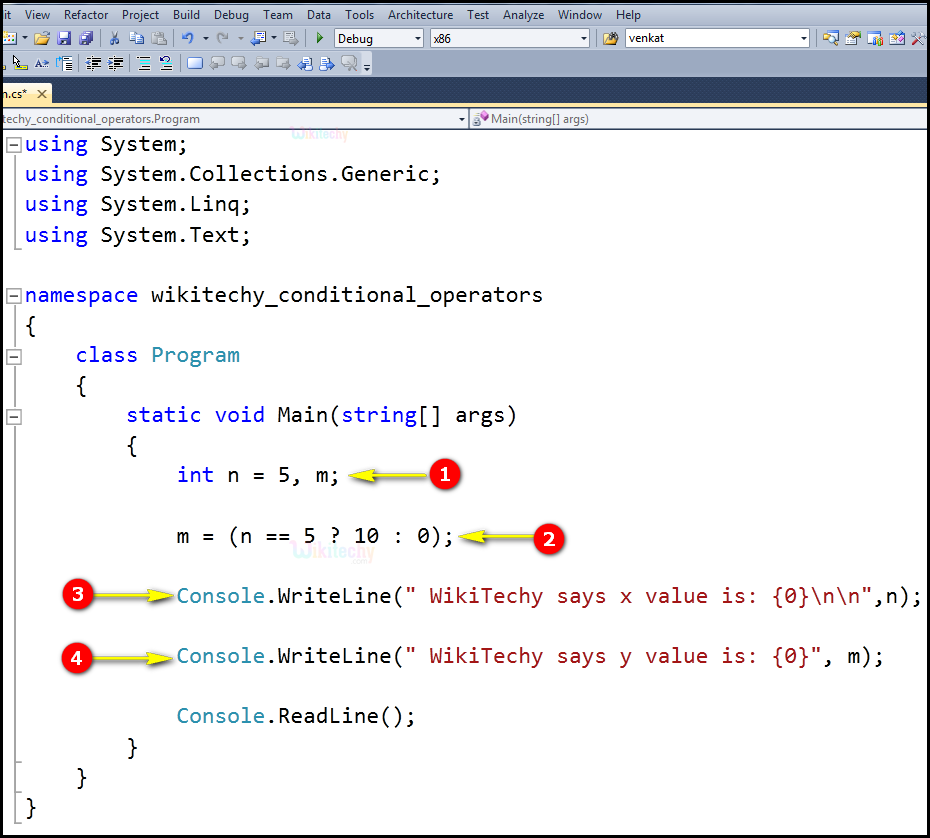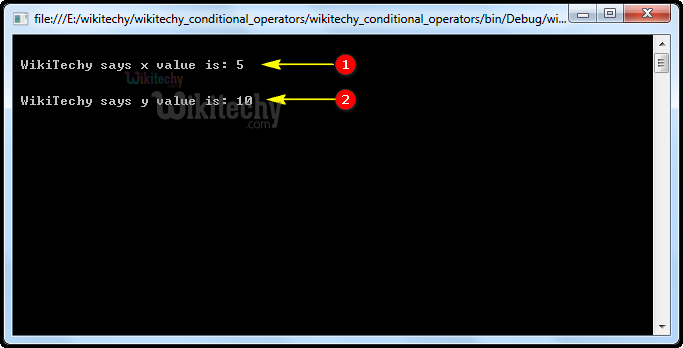# C# Conditional Operators - c# - c# tutorial - c# netC# Conditional Operators

## What is the use of Conditional Operators in C# ?

• Conditional operators return one value if condition is true and returns another value is condition is false.
• Conditional operators are also known as ternary operator represented by the symbol " ? :" (question mark).
• Conditional operators are also called as ternary operator.Conditional Operator

## Code Explanation:1. In this statement, we declare the variable "n=5" and m as integer.
2. In this statement, we check the condition using ternary operator that is if "n==5" means it sets "true", so the value for "y" is "10" otherwise it sets the "y" value as "0".
3. Here we print the value of "n" using the "WikiTechy says x value is:" statement.
4. Here we print the value of "m" using the "WikiTechy says y value is:" statement.

## Sample C# examples - Output :1. Here we printed the value of "WikiTechy says x value is:" as "5".
2. Here in this output the conditional operation has been performed and the condition has been satisfied so the value of the "WikiTechy says x value is:" variable will be printed as "10".